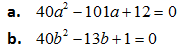New Student User - Use Code HELLO

### Question 1.

In the following question two equations (A) and (B) given. You have to solve bothi. If a>b
ii. If a≤b
iii. If a=b
iv. If a<b
v. If a≥b
In the following question two equations (A) and (B) given. You have to solve both:
In the following question two equations (A) and (B) given. You have to solve both:
In the following question two equations (A) and (B) given. You have to solve both:
In the following question two equations (A) and (B) given. You have to solve both:
In the following question two equations (A) and (B) given. You have to solve both:
In the following question two equations (A) and (B) given. You have to solve both:
In the following question two equations (A) and (B) given. You have to solve both: